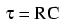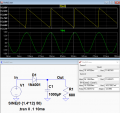# Half Wave Rectifier ripple voltage?

#### 2nowman

Joined Jan 14, 2017
17
Hello,

But i've been trying to do some theoretical calculations for a half wave rectifier... the circuit is a 12V-0V-12V AC power supply at 50hz, a 1N4001 diode with 680ohm resistor as the load, then a 1000uF capacitor in parallel with the resistor providing some smoothing.

I'm trying to find the voltage peak to peak of the ripple that results, but i think i need the average dc current of the ripple? once i have that, i was planning to use the formula; changeinVoltage = current(dc) / frequency x capacitance, so up to now i've got;
changeinVoltage = I(dc) / 50hz x 1000uF, so it's just the current i'm missing.

If anyone can point me in the right direction on how to work this out that would be great, i've been searching the internet for ages, and don't seem to be getting anywhere.

PS i'm new to electronics and the maths behind it, so please go easy on me.

#### ericgibbs

Joined Jan 29, 2010
11,584

#### 2nowman

Joined Jan 14, 2017
17
Thanks for the reply, but i've read a few things like that and still dont understand, i understand what is happening, but i'm not good enough with the maths - think it's time to give up.

Thanks again.

#### ericgibbs

Joined Jan 29, 2010
11,584
hi 2,
Don't give up, just ask specific questions and we will try to talk you thru the problem.
E

#### crutschow

Joined Mar 14, 2008
25,995
A simple approximation is to assume the capacitor charges to the peak of the AC waveform (minus the diode drop) and the capacitor voltage decays after that with the RC time-constant of the load resistance and the filter capacitor value until the next peak.
The formula for that iswhere t the time andis the time constant (680 * 1000uf = 680ms for your values).
Thus, for 50Hz, t=20ms so plugging those two values into the formula gives a percentage change of 2.90%.

For a 12Vac signal the peak voltage is 1.4*12V = 16.8V, thus the capacitor will charge to about 16.8V - 0.7V = 16.1V.
The decay (ripple) voltage is thus approximately 16.1V x 2.9% = 0.466V between peaks.
(That's a worst-case value since the capacitor will actually start to charge back up slightly before the next peak, but it's close enough for most design work).

For an even simpler calculation you could use the average current of the load, as you suggested.
Assuming the ripple voltage is low we can use the value of 16V / 680 = 23.5mA as an approximation for the average current.
This gives a voltage (ripple) decay of (23.5mA*20ms) / 1000uF = .0.47V, a little higher, but close to the above calculation.

Below is an LTspice simulation of the circuit, which gives a ripple value of 0.434V, slightly below both the calculated values as expected.Last edited:

#### dannyf

Joined Sep 13, 2015
2,197
I'm trying to find the voltage peak to peak of the ripple that results,
it is difficult to do exact calculation but good estimation fairly easy.

1) the peak voltage is 1.4 * 12v = ~15v for easier math.
2) after that, the capacitor will discharge through the resistor for just shy of 1 period for half wave rectification. for a 1000uf cap + 680R for (1/50hz) = 20ms, the decline from the peak would follow exp(-t/rc), or in this case, about 3%, or 0.5v.

the rest is fairly easy to calculate.

you can further refine the analysis, for example, by incorporating the forward voltage drop on the diode, ...